## One Dimensional Kinematics: Extra Problems

The problems below are provided as additional problems to the original set of 35 Kinematics problems. Just like problems in the original set, these problems consist of a problem, an answer (revealed by clicking a link), an audio guided solution, and links to an Overview page of formulas and to The Physics Classroom Tutorial pages. The Extra Problems pertain to various aspects of the topic of kinematics concepts (such as distnace, displacement, speed, velocity and acceleration), kinematic graphing, and kinematic equations. The problems are divided into five separate sets of problems. The first set pertains to kinematic concepts. The second set focuses on the use of position-time graphs. The third set focuses on the use of velocity-time graphs. The last two sets pertain to the use of kinematic equations.

### Problem Set A

Problem 1:

A football player makes a touch down run for 93.4 yards in a time of 14.3 seconds. What was his average velocity in m/s? (Given: 39.7 in = 1.00 m)

View Audio Solution.

Problem 2:

If the average speed of an orbiting space shuttle is 19600 miles per hour, find the time (in hours) required for it to circle the earth. Use the distance around the earth in your calculation and neglect the fact that the shuttle is about 205 miles above the earth's surface. (GIVEN: 1 m/s = 2.24 mi/h; Earth radius = 6.36 x 106 m.)

Problem 3:

A certain bacterium swims with a speed of 4.22 mm/s. How much time (in s) would it take this bacterium to swim across a petri dish having a diameter of 9.35 cm?

Problem 4:

A jogger runs eastward in a straight line with an average speed of 3.39 m/s for 5.4 min and then continues with an average speed of 2.05 m/s for 2.1 min. What is her total displacement (in m)?

Problem 5:

(Referring to the previous problem - #4) What is her average velocity (in m/s) during this entire jog?

Problem 6:

Mr. H waits patiently as two beetles race across the 35.70-cm length of the Wheaties box. Beetle A averages 3.77 mm/s and Beetle B averages 5.33 mm/s. If Beetle A gets a 11.76-cm 'head start' (when Beetle B is at the far edge of the box), then what is the separation distance (in cm) between beetles when the winning beetle reaches the end of the box? Calculate your answer accurate to the second decimal place.

Problem 7:

An athlete swims the length of a 52.8-m pool in 21.2 s and makes the return trip to the starting position in 23.7 s. Determine her average velocity (in m/s) in the first half of the swim.

Problem 8:

(Referring to the previous problem.) Determine her average velocity (in m/s) for the entire round trip.

Problem 9:

A person takes a trip, driving with a constant speed of 93.9 km/h except for a 21.6-min rest stop. If the person's average speed is 79.9 km/h, for how much time (in minutes) was the person on the road (i.e., traveling as opposed to resting)?

Problem 10:

Determine the acceleration (in m/s/s) of a car which can accelerate from 0 mi/h to 57.3 mi/h in 5.38 seconds. (Given: 1.00 m/s = 2.24 mi/h)

Problem 11:

A car traveling in straight line has a velocity of +6.3 m/s at some instant. After 9.11 s, its velocity is equal to +25.5 m/s. What is its average acceleration (in m/s/s) in this time interval? Indicate the direction using a + or a - sign.

Problem 12:

A car traveling in a straight-line path has a velocity of +24.4 m/s at some instant. After 7.48 s, its velocity is +6.4 m/s. What is its average acceleration (in m/s/s) during this time interval? Indicate the direction using a + or a - sign.

Problem 13:

A tennis ball with a speed of 11.9 m/s is thrown perpendicularly at a wall. After striking the wall, the ball rebounds in the opposite direction with a speed of 7.5 m/s. If the ball is in contact with the wall for 0.012 s, what is the average acceleration (in m/s/s) of the ball while in contact with the wall? Enter a positive answer only!

Problem 14:

A car traveling initially at +8.18 m/s accelerates at the rate of 0.870 m/s/s for a time of 9.03 s. What is its velocity (in m/s) at the end of the acceleration?

Problem 15:

A certain car is capable of accelerating at a rate of +4.00 m/s/s. How long a time (in seconds) is taken for this car to go from a speed of 46.8 mi/h to a speed of 67.9 mi/h? (Given: 1 m/s = 2.24 mi/h)

### Problem Set B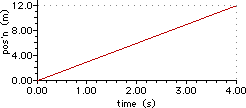Problem 1:

Consider the position-time graph at the right. Determine the velocity (in m/s) of the object whose motion is represented by the graph. Indicate the direction using a + or a - sign.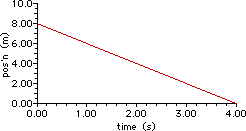Problem 2:

Consider the position-time graph at the right. Determine the velocity (in m/s) of the object whose motion is represented by the graph. Indicate the direction using a + or a - sign.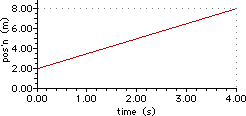Problem 3:

Consider the position-time graph at the right. Determine the velocity (in m/s) of the object whose motion is represented by the graph.Indicate the direction using a + or a - sign.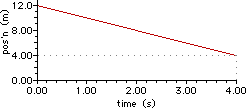Problem 4:

Consider the position-time graph at the right. Determine the velocity (in m/s) of the object at 3 seconds. Indicate the direction using a + or a - sign.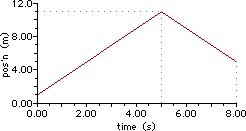Problem 5:

Consider the position-time graph at the right. Determine the velocity (in m/s) of the object during the first 4 seconds. Indicate the direction using a + or a - sign.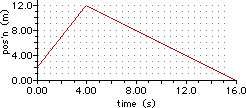Problem 6:

Consider the position-time graph at the right. What is the net displacement (in m) of the object after 13 seconds? Indicate the direction using a + or a - sign.Problem 7:

Consider the position-time graph at the right. What is the total distance (in m) which the object has traveled after 13 seconds?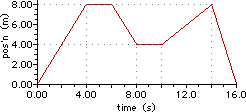Problem 8:

Consider the position-time graph at the right. Determine the distance traveled (in m) by the object after 15 seconds.Indicate the direction using a + or a - sign.Problem 9:

Consider the position-time graph at the right. Determine the greatest velocity (in m/s) obtained by this object (consider magnitude only in determining 'greatness'). Indicate the direction using a + or a - sign.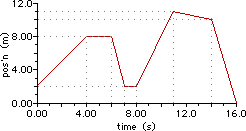Problem 10:

Consider the position-time graph at the right. At what time (in seconds) does the object have the greatest displacement?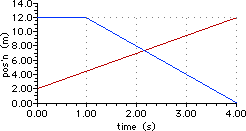Problem 11:

Consider the position-time graph at the right. The red line represents object A's motion and the blue line represents object B's motion. How much faster (in m/s) is the faster of the two objects moving compared to the slower object at a time of 3.7 seconds?Problem 12:

Consider the position-time graph at the right. Determine the average velocity (in m/s) of the object for the entire 16 seconds of motion. Indicate the direction using a + or a - sign.Problem 13:

Consider the position-time graph at the right. Determine the average velocity (in m/s) of the object for the first 13 seconds of motion. Indicate the direction using a + or a - sign.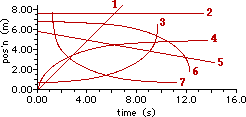Problem 14:

Consider the position-time graphs shown at the right. The motion of seven objects is depicted on the graph. Which of the objects is/are speeding up?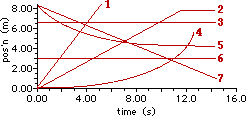Problem 15:

Consider the position-time graph shown at the right. The motion of seven objects is depicted on the graph. On average, which one of the objects is moving with the greatest magnitude of velocity?

### Problem Set C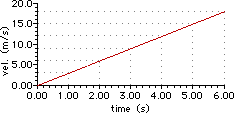Problem 1:

Consider the velocity-time graph at the right. Determine the acceleration (in m/s/s) of the object whose motion is represented by the graph. Indicate the direction using a + or a - sign.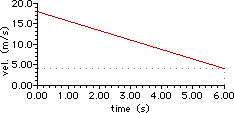Problem 2:

Consider the velocity-time graph at the right. Determine the acceleration (in m/s/s) of the object whose motion is represented by the graph. Indicate the direction using a + or a - sign.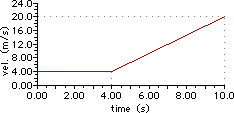Problem 3:

Consider the velocity-time graph at the right. Determine the acceleration (in m/s/s) of the object at 8.9 seconds. Indicate the direction using a + or a - sign.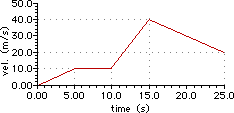Problem 4:

Consider the velocity-time graph at the right. Determine the acceleration values (in m/s/s) of the object at 2.9 seconds, at 13.9 seconds, and at 23.8 seconds. Indicate the direction using a + or a - sign.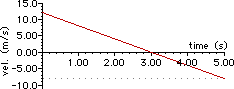Problem 5:

Consider the velocity-time graph at the right. Determine the acceleration (in m/s/s) of the object at a time of 3.0 seconds. Indicate the direction using a + or a - sign.Problem 6:

Consider the velocity-time graph at the right. Determine the displacement (in m) of the object after a time of 5 seconds. Indicate the direction using a + or a - sign.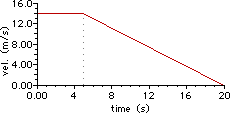Problem 7:

Consider the velocity-time graph at the right. Determine the displacement (in m) of the object during the entire 20 seconds of motion. Indicate the direction using a + or a - sign.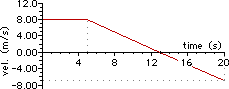Problem 8:

Consider the velocity-time graph at the right. Determine the displacement (in m) of the object during the entire 20 seconds of motion. Indicate the direction using a + or a - sign.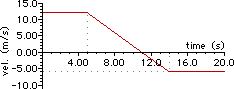Problem 9:

Consider the velocity-time graph at the right. Determine the displacement (in m) of the object during the first 19 seconds of motion. Indicate the direction using a + or a - sign.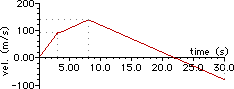Problem 10:

The velocity-time graph at the right depicts the motion of a two-stage rocket. At what time (in seconds) does the rocket change its direction and begin to fall from its 'peak'?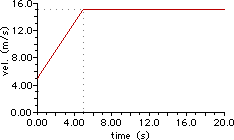Problem 11:

Consider the velocity-time graph at the right. Determine the displacement (in m) of the object during the first 18 seconds of motion. Indicate the direction using a + or a - sign.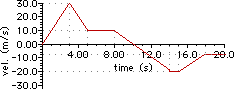Problem 12:

Consider the velocity-time graph at the right. Identify all the times (in seconds) that the object made a direction change.Problem 13:

Consider the velocity-time graph at the right. Identify all the acceleration values (in m/s/s) for the various sections of the graph.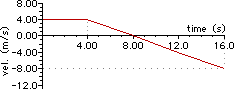Problem 14:

The velocity-time graph at the right depicts an object which starts moving in one direction and turns around and moves in the other direction. Determine the time (in seconds) at which the object reaches its initial position (i.e., starting point). (HINT: develop an equation for displacement based on the slope of the line and compute the unknown.)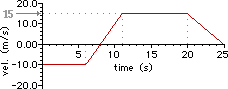Problem 15:

Consider the velocity-time graph at the right. Identify all the acceleration values (in m/s/s) for the various sections of the graph.Problem 16:

Consider the velocity-time graph at the right. Determine the displacement (in m) of the object after 24 seconds.Indicate the direction using a + or a - sign.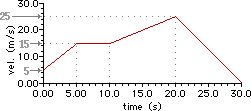Problem 17:

Consider the velocity-time graph at the right. Determine the displacement (in m) of the object after 28 seconds. Indicate the direction using a + or a - sign.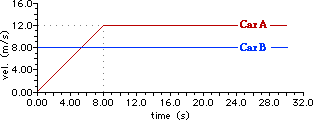Problem 18:

Consider the velocity-time graph for Car A (red line) and Car B (blue line). At what time (in seconds) is Car A ahead of Car B by a distance of 71 meters?

Problem 19: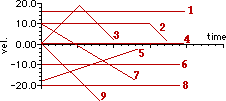Consider the velocity-time graph at the right for several different objects, each represented by a numbered line. List the numbers of any and all objects which is/are slowing down as it moves in the negative direction at any time during the course of their motion.

Problem 20: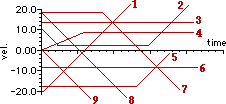Consider the velocity-time graph at the right for several different objects, each represented by a numbered line. List the numbers of any and all objects which is/are slowing down as it moves in the negative direction at any time during the course of their motion.

### Problem Set D

Problem 1:

A speedboat increases its speed from 23.8 m/s to 34.2 m/s in a distance of 231 m. Determine the acceleration (in m/s/s) of the speedboat.

View Audio Solution.

Problem 2:

(Referring to the previous problem.) Determine the time (in s) over which this acceleration occurs.

View Audio Solution.

Problem 3:

A racing car reaches a speed of 48.4 m/s. At this instant, it begins a uniform deceleration using a parachute and a braking system and comes to rest 5.76 s later. Determine the acceleration (in m/s/s) of the car. Indicate the direction with a + or - sign.

View Audio Solution.

Problem 4:

(Referring to the previous problem.) Determine the displacement (in m) of the racing car during this deceleration period.

View Audio Solution.

Problem 5:

A jet plane lands with a velocity of +116 m/s and can accelerate at a maximum rate of -6.73 m/s/s as it comes to rest. From the instant it touches the runway, what is the minimum time (in seconds) needed before it comes to rest?

View Audio Solution.

Problem 6:

A car, initially traveling at 24.0 m/s, accelerates at a uniform rate of 5.68 m/s/s for a distance of 57.5 m. How much time (in seconds) is required to cover this distance?

View Audio Solution.

Problem 7:

A 438-m long train is moving on a straight track with a speed of 87.7 km/hr. The engineer applies the brakes at a crossing and later the caboose passes the crossing with a speed of 19.0 km/hr. Assuming constant acceleration, determine the length of time (in seconds) that the train blocks the crossing. Disregard the width of the crossing.

View Audio Solution.

Problem 8:

In order to pass a physical education class at a university, a student must run 1 mile in 12.0 minutes. After running for 10.0 minutes at a constant pace, she still has 581 yds to go. Assuming that she accelerates uniformly over the remainder of the run, what acceleration (in m/s/s) would she need to pass the test? (GIVEN: 1 mi = 1609 m; 3.28 ft = 1.00 m).

View Audio Solution.

Problem 9:

A 72-kg hockey player is standing on his skates on a frozen pond when an opposing player moving with a uniform speed of 13.5 m/s skates by with the puck. After 3.37 s, the first player makes up his mind to chase his opponent. If he accelerates uniformly at 5.69 m/s/s, how much time (in seconds) does it take him to catch his opponent? (Assume that the player with the puck remains in motion at constant speed.)

View Audio Solution.

Problem 10:

(Referring to the previous problem.) What distance (in meters) will the first player (the one that is accelerating) move before catching up with the opposing player?

View Audio Solution.

Problem 11:

A bicyclist starts down a hill with an initial speed of 2.83 m/s. She moves down the hill with a constant acceleration, arriving at the bottom with a speed of 10.6 m/s. If the hill is 15.2 m long, then what is the acceleration (in m/s/s) of the bicyclist on the hill?

View Audio Solution.

Problem 12:

A blue car is at rest at a stoplight. The moment the stoplight turns green, a red car passes it moving with a constant speed of 21.1 m/s. The blue car accelerates uniformly at a rate of 9.24 m/s/s. How much time (in seconds) will be required for the blue car to catch up with the red car?

View Audio Solution.

Problem 13:

A green car is following a yellow car down an expressway at a constant speed of 77.8 mi/hr. The separation distance between the two cars is 38.7 ft. The yellow car slams on the brakes and decelerates at a rate of -8.85 m/s/s. After a reaction of time of .381 seconds, the green car begins decelerating at a rate of -8.69 m/s/s. What is the final separation distance (in m) between the cars once stopped? (Given: 1 m/s = 2.24 mi/hr; 3.28 ft = 1 m)

View Audio Solution.

Problem 14:

A train normally travels with a uniform speed of 88 km/hr on a long stretch of straight, level track. On a particular day, the train must make a 3.42 minute stop at a station along this track. If the train decelerates at a uniform rate of -1.87 m/s/s and accelerates at a rate of 0.75 m/s/s, then how much time (in minutes) is lost by the train in making the stop.

View Audio Solution.

Problem 15:

A military aircraft is launched from an aircraft carrier along a 92.3-m runway. It is accelerated from rest for 5.32 seconds. Determine the launch speed (in m/s) of the plane.

View Audio Solution.

### Problem Set E

Problem 1:

A ball is thrown vertically upwards with a speed of 41.1 m/s. How high (in meters) does it rise above the point of release?

View Audio Solution.

Problem 2:

(Referring to the previous problem.) How much time (in seconds) does it take the ball to reach the peak of its trajectory?

View Audio Solution.

Problem 3:

A pebble is dropped into a deep water well, and the sound of the splash as the pebble reaches the bottom of the well is heard 4.63 seconds later. From this information, estimate the depth (in meters) of the well. (Neglect the time required for the sound to travel the length of the well.)

View Audio Solution.

Problem 4:

When a nurse squeezes a syringe, the liquid squirts 51.7 cm into the air. With what speed (in cm/s) does the liquid emerge from the syringe?

View Audio Solution.

Problem 5:

A juggler performs in a room with a ceiling 2.79 m above hand level. What is the maximum upward speed (in m/s) which she can give a ball without letting it hit the ceiling?

View Audio Solution.

Problem 6:

A peregrine falcon dives at a pigeon. The falcon starts downward from rest and falls with the acceleration of gravity. If the pigeon is 85.3 m below the falcon, how long (in seconds) does it take the falcon to reach the pigeon?

View Audio Solution.

Problem 7:

A parachutist descending at a speed of 18.3 m/s drops a camera from an altitude of 65.8 m. What is the speed (in m/s) with which the camera strikes the ground?

View Audio Solution.

Problem 8:

A women is reported to have fallen 52.2 m from the 17th floor of a building and to have landed on a metal ventilator box, which she crushed to a depth of 52.8 cm. She suffered only minor injuries. Neglecting air resistance, calculate the speed (in m/s) of the woman just before she collided with the box.

View Audio Solution.

Problem 9:

A basketball player has a vertical leap of 1.26 m. Determine the speed (in m/s) at which the player leaves the ground in order to reach this vertical height?

View Audio Solution.

Problem 10:

A biker speeds up to 32.4 m/s from rest in 6.48 seconds. The biker maintains this speed for 7.01 seconds before coasting to a stop in 6.00 seconds. What was the biker's average speed (in m/s) for the trip?

View Audio Solution.

Problem 11:

An arrow is shot upward at 64.8 m/s. What is its average speed (in m/s) during the third second of its flight? Ignore air resistance.

View Audio Solution.

Problem 12:

A two-stage rocket is launched straight up from rest. It accelerates at 27.8 m/s/s for 3.74 seconds during the first stage. It accelerates at 18.5 m/s/s for 5.60 more seconds during the second stage. After second stage burnout the rocket comes under the influence of gravity only. Determine its height above launch point (in meters) after 25.19 seconds from launch.

View Audio Solution.

Problem 13:

On the 4th of July fireworks are being shot straight up into the air with a launch velocity of 51.9 m/s. What is the earliest time (in seconds) when the fireworks will be 46.0 meters above the ground? Ignore air resistance.

View Audio Solution.

Problem 14:

A red car moving at 61.9 mph passes a blue car moving at 37.3 mph. (Use 1609 meters = 1 mile when converting to meters/second.) Seeing a stopped car in his lane up ahead, the driver of the red car applies the brakes just as he passes the blue car which causes the red car to slow down to a stop by losing 4.67 m/s every second. How much time (in seconds) passes before the two cars are side-by-side once again?

View Audio Solution.

Problem 15:

A red car is sitting at a stop light at rest. When the light turns green two things happen simultaneously; (1) the red car accelerates to a speed of 32.7 m/s in 8.48 seconds and then maintains that 32.7 m/s speed and (2) a blue car passes by the red car at a constant speed of 21.4 m/s. How much time (in seconds) will have elapsed from when the light turned green until the two cars are side-by-side?

View Audio Solution.